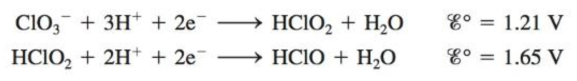Chapter 20, Problem 84AE

Chapter
Section
Textbook Problem

# What is a disproportionation reaction? Use the following reduction Potentialsto predict whether HClO2 will disproportionate.

Interpretation Introduction

Interpretation: Disproportionation reaction is to be defined. If HClO2 will disproportionate or not in the given reduction potentials is to be predicted.

Concept introduction: The oxidation and reduction of an element simultaneously in a reaction is known as disproportionation reaction.

If the value of electrode potential of cell is positive, the molecule will disproportionate in the reaction whereas, if the value of electrode potential of cell is negative, the molecule will not disproportionate in the reaction.

To determine: The proper definition of disproportionation reaction; if HClO2 will disproportionate in the given reduction potentials.

Explanation

The oxidation and reduction of an element simultaneously in a reaction is known as disproportionation reaction.

Disproportionation means oxidation and reduction of an element simultaneously in a reaction. It is a type of redox reaction. In this reaction, reactant molecule produces two or more dissimilar products.

Yes, HClO2 will disproportionate in the given reduction potentials.

Given

Electrode potential at anode is,

ClO3+3H++2eHClO2+H2O

The electrode potential for the above reaction is 1.21V .

Electrode potential at cathode is,

HClO2+2H++2eHClO+H2O

The electrode potential for the above reaction is 1.65V .

The oxidation half reaction for the given reduction potentials are,

HClO2(aq)+H2O(aq)ClO3(aq)+3H+(aq)+2e

The reduction half reaction for the given reduction potentials are,

HClO2(aq)+2H+(aq)+2eHClO(aq)+H2O(l)

Cell reaction is the combination of oxidation half reaction and reduction half reaction

### Still sussing out bartleby?

Check out a sample textbook solution.

See a sample solution

#### The Solution to Your Study Problems

Bartleby provides explanations to thousands of textbook problems written by our experts, many with advanced degrees!

Get Started